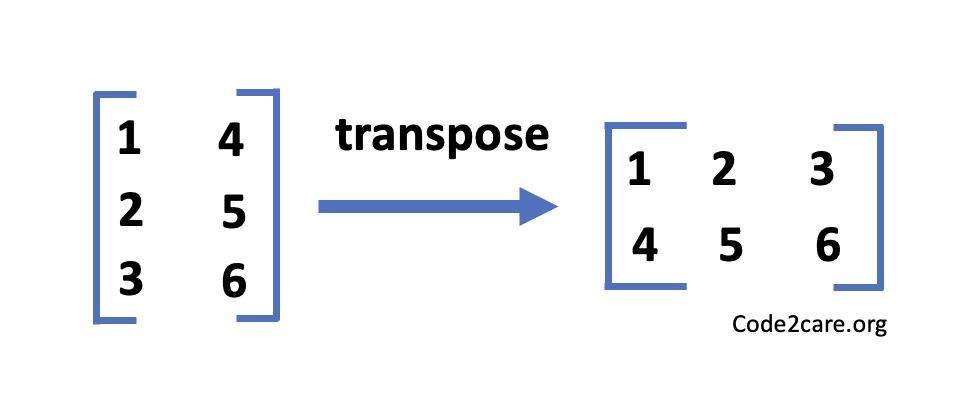# How to Transpose a Square Matrix - Java Program

The transposition of a matrix is one of the most commonly studied questions in school or collages when learning Java Programs in practical labs.In the transposition of a matrix we are supposed to flip the matrix over its diagonal, i.e. the rows of the original matrix become the columns and the columns of the original matrix become the rows of the transposed matrix.

## Pseudo Logic Building

1. First we declare a 2-dimensional array to represent a matrix.
2. We add the values to the elements of the array.
3. We find the dimension of the matrix - rows and column size.
4. Create a new array (matrix) of the swapped dimensions - rows of our original array becomes columns and columns as row.
5. Finally we loop the rows and columns of the original matrix and add these elements to the column and row of the transposed matrix.
6. Finally we print our transposed matrix.

## Java Program To Transpose A Matix

``````package org.code2care.java.arrays.examples;

import java.util.Arrays;

/**
* Java Program to transpose a
* Matrix
* <p>
* Author: Code2care.org
* Date: 28 Apr 2023
*/
public class JavaMatrixTranspose {

public static void main(String[] args) {

//2-D Matrix
int[][] originalMatrix = {
{1, 4},
{2, 5},
{3, 6}
};

int matrixRows = originalMatrix.length;
int matrixCols = originalMatrix.length;

//New matrix to store the transposed rows and columns
int[][] transpose = new int[matrixCols][matrixRows];

for (int i = 0; i < matrixRows; i++) {
for (int j = 0; j < matrixCols; j++) {
//copy into transposed originalMatrix
transpose[j][i] = originalMatrix[i][j];
}
}

System.out.println("Input Matrix:");
Arrays.stream(transpose)
.forEach(row -> {
Arrays.stream(row)
.forEach(element -> System.out.print(element + " "));
System.out.println();
});

System.out.println("Transposed Matrix:");
Arrays.stream(originalMatrix)
.forEach(row -> {
Arrays.stream(row)
.forEach(element -> System.out.print(element + " "));
System.out.println();
});

}
}
``````

Output:

``````Input Matrix:
1 2 3
4 5 6

Transposed Matrix:
1 4
2 5
3 6 ``````

Let us see another example with a Square 3x3 matix.

## Tranpose a 3x3 Matix in Java

``````package org.code2care.java.arrays.examples;

import java.util.Arrays;

/**
* Transpose 3x3 Matrix
* Author: Code2care.org
* Date: 28 Apr 2023
*/
public class JavaMatrixTranspose {

public static void main(String[] args) {

//3x3 Matrix
int[][] input3x3Matrix = {
{21, 14, 41},
{23, 53, 34},
{43, 46, 56}
};

int matrixRows = input3x3Matrix.length;
int matrixCols = input3x3Matrix.length;

int[][] transpose = new int[matrixCols][matrixRows];

for (int i = 0; i < matrixRows; i++) {
for (int j = 0; j < matrixCols; j++) {
transpose[j][i] = input3x3Matrix[i][j];
}
}

System.out.println("Input Matrix:");
Arrays.stream(transpose)
.forEach(row -> {
Arrays.stream(row)
.forEach(element -> System.out.print(element + " "));
System.out.println();
});

System.out.println("Transposed Matrix:");
Arrays.stream(input3x3Matrix)
.forEach(row -> {
Arrays.stream(row)
.forEach(element -> System.out.print(element + " "));
System.out.println();
});

}
}
``````

Output:

``````Input Matrix:
21 23 43
14 53 46
41 34 56

Transposed Matrix:
21 14 41
23 53 34
43 46 56 ``````
-

Have Questions? Post them here!